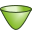# HY Card

With this card a hyperboloid section can be created.

On the Construct tab, in the Surfaces group, click theHyperboloid (HY) icon.

## Parameters:

S1
The origin (point at which the asymptotes intersect) of the hyperboloid section.
S2
The pole of the hyperbolic border.
S3
A point that defines the outer border of the hyperboloid section.
Normal vector directed
The normal vectors can be directed Inward or Outward.
Subtended angle $\phi$ (in degrees):
The subtended angle measured from S3.
Max. triangle edge length (at circular border):
The maximum edge length on the circular border of the hyperboloid section.

The hyperbolic border may require shorter mesh edges than those used for straight edges. Therefore the maximum segment length specified in the IP card may be overridden along the circular arc by setting Max. triangle edge length (at circular border).

## Example of HY card usage:

The hyperboloid section shown is created using the HY card.